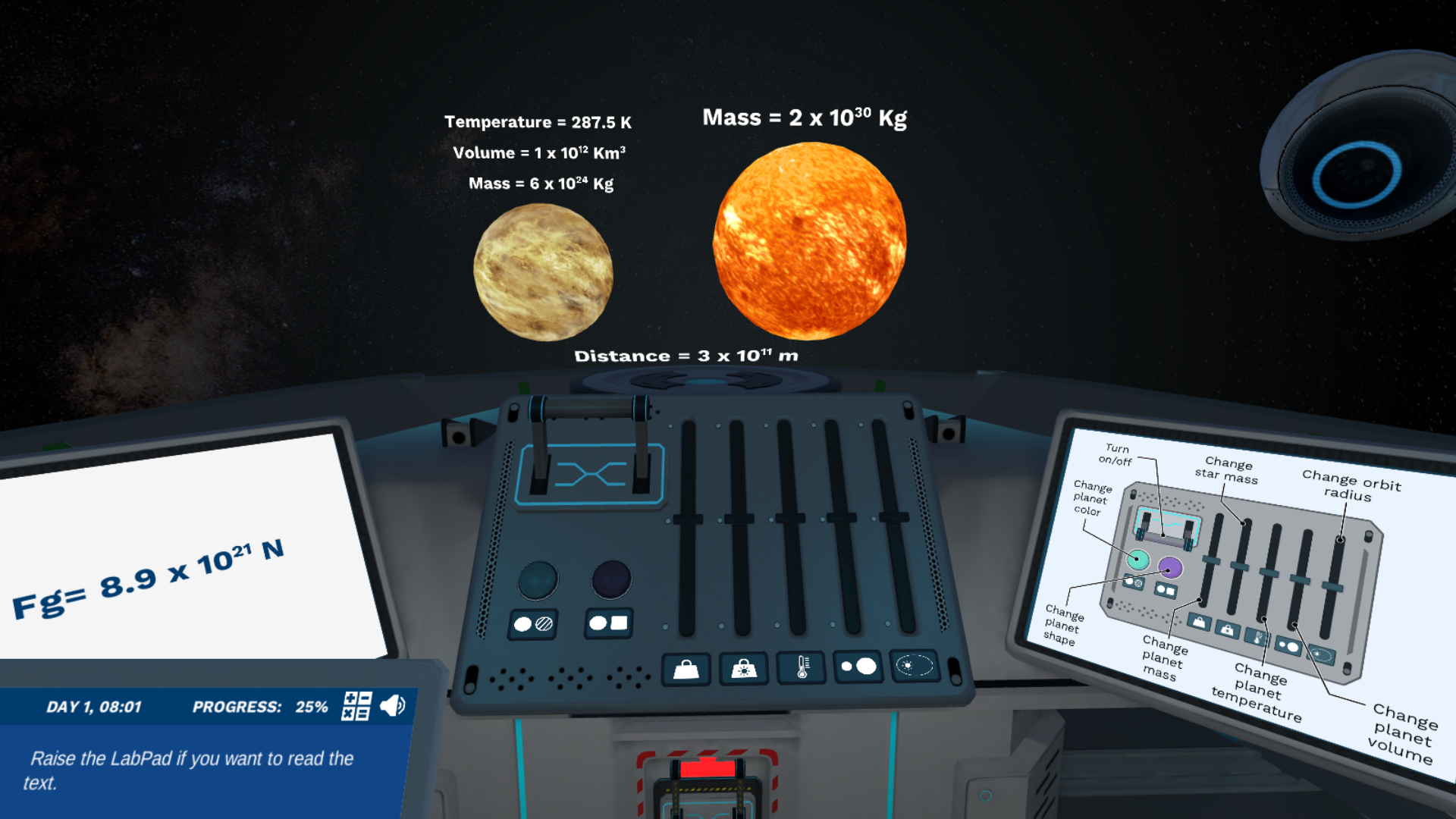Back to simulations

# Newton's Law of Gravitation: Mathematical expression of gravitational force Virtual Lab

Join Newton in his garden and take a spaceship ride to explore gravity in space. Learn the meaning of each term of the equation of the universal law of gravitation and use it to predict the gravitational attraction between two bodies.High SchoolPlaying with planets and stars has never been so easy. Dr. One will welcome you to a virtual lab on a spaceship where you’ll have high-tech buttons to play with. They’ll allow you to change the properties of celestial bodies with a simple click and visualize the effect of each term of the gravitational equation. But that’s not all. Have you ever wondered what would happen if the gravitational constant was not part of the equation or why planets don't fall into the Sun? Press play and find out.

Let’s go to space with Newton and understand his law of universal gravitation

The story goes that it was an apple that gave Newton his idea of ​​universal gravitation. What if the gravitational force was not just affecting just apples or terrestrial objects, but also causing planetary motion? Luckily, we can go to space with our spaceship and find out. Up there, we will change the properties of a planet and a star to understand what affects the gravitational force. Afterwards, we will play a card game to build up the gravitational equation and test it on Earth.

Let’s investigate how the terms of the equation are related

Once we identify WHICH variables affect the gravitational attraction between two bodies, we’ll investigate HOW they affect it. In particular, we will play a card game and use graphs to identify the proportionality between the gravitational force and the terms of the gravitational equation: the masses of two objects and their distance.

Time to try our gravitational equation on Earth

Let’s go back to Earth and test if our equation can describe the gravitational attraction between two objects. Here on Earth, we will learn how the gravitational force would look like without the gravitational constant in the equation. With the support of Dr. One and Newton we will understand how important G is.

Also, have you ever shot someone into space with a cannon? If not, playing this simulation will allow you to do it. We will set up the perfect experiment to make possible what Newton had only imagined. We will test if his law of universal gravitation is also responsible for planetary motion.

#### Learning Objectives

At the end of this simulation, you will be able to:

• Identify the components of gravitational force

• Explain how the gravitational force is related to the masses of two objects and the distance between them

• Use the gravitational formula to calculate the gravitational force between two distant objects

#### Techniques in Lab

At the end of this simulation, you will be able to:

• Equation of the gravitational force
• Terms of an equation
• Direct and inverse proportionality
• Gravitational constant

## How do virtual labs work?

Engage students in science through interactive learning scenarios. Simulate experiments, train lab techniques, and teach theory through visual experiences that enhance long-term learning outcomes.300+ Web-based simulations that can be played on laptops, Chromebooks, and tablets/iPads without installing any softwareTeacher dashboard to automate grading and track student progressEmbedded quizzes to help students master science contentLibrary of learning resources, lab reports, videos, theory pages, graphics and more

### Get Started Now!

Try Labster with your students right away.Track student progressAssess with customizable quizzesInvite your students to play simulationsExplore over 300 Labster simulations